# Delta to Star Conversion Notes | Study Network Theory (Electric Circuits) - Electrical Engineering (EE)

## Electrical Engineering (EE): Delta to Star Conversion Notes | Study Network Theory (Electric Circuits) - Electrical Engineering (EE)

The document Delta to Star Conversion Notes | Study Network Theory (Electric Circuits) - Electrical Engineering (EE) is a part of the Electrical Engineering (EE) Course Network Theory (Electric Circuits).
All you need of Electrical Engineering (EE) at this link: Electrical Engineering (EE)

Delta Network

Consider the following delta network as shown in the following figure.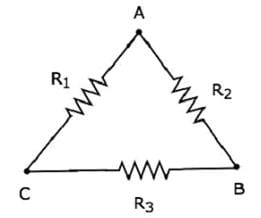The following equations represent the equivalent resistance between two terminals of delta network, when the third terminal is kept open.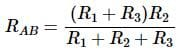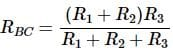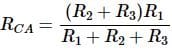Star Network

The following figure shows the equivalent star network corresponding to the above delta network.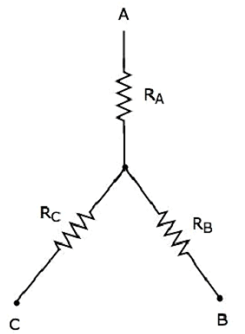The following equations represent the equivalent resistance between two terminals of star network, when the third terminal is kept open.
RAB = RA + RB
RBC = RB + RC
RCA = RC + RA

Try yourself:Star connection is also known as ________.

Conversion of Star Network Resistances into Delta Network Resistances

• We will get the following equations by equating the right-hand side terms of the above equations for which the left-hand side terms are same.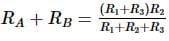Equation 1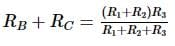Equation 2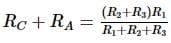Equation 3
• By adding the above three equations, we will get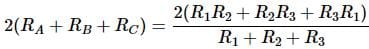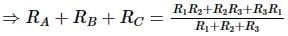Equation 4
• Subtract Equation 2 from Equation 4.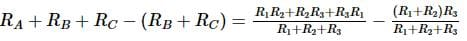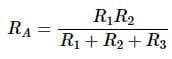• By subtracting Equation 3 from Equation 4, we will get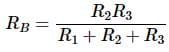• By subtracting Equation 1 from Equation 4, we will get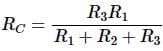• By using the above relations, we can find the resistances of star network from the resistances of delta network. In this way, we can convert a delta network into a star network.

Example.1 Let us calculate the resistances of star network, which are equivalent to that of delta network as shown in the following figure.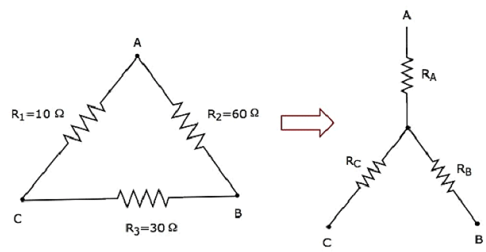• Given the resistances of delta network as R1 = 10 Ω, R2 = 60 Ω and R3 = 30 Ω.
• We know the following relations of the resistances of star network in terms of resistances of delta network.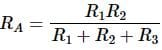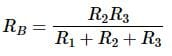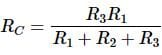• Substitute the values of R1, R2 and R3 in the above equations.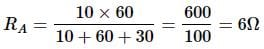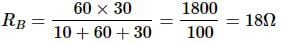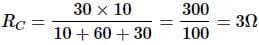• So, we got the resistances of star network as RA = 6 Ω, RB = 18 Ω and RC = 3 Ω, which are equivalent to the resistances of the given delta network.

Try yourself:The value of the 3 resistances when connected in star connection is ________.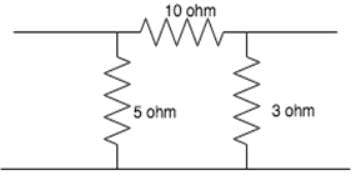The document Delta to Star Conversion Notes | Study Network Theory (Electric Circuits) - Electrical Engineering (EE) is a part of the Electrical Engineering (EE) Course Network Theory (Electric Circuits).
All you need of Electrical Engineering (EE) at this link: Electrical Engineering (EE)Use Code STAYHOME200 and get INR 200 additional OFF

## Network Theory (Electric Circuits)

23 videos|21 docs|27 tests

Track your progress, build streaks, highlight & save important lessons and more!

,

,

,

,

,

,

,

,

,

,

,

,

,

,

,

,

,

,

,

,

,

;